Sunday, August 9, 2020
Home > CBSE Class 12 > NCERT Solutions for Class 12 Maths Determinants Exercise 4.5

# NCERT Solutions for Class 12 Maths Determinants Exercise 4.5# NCERT Solutions for Class 12 Maths Determinants

Hi Students, Welcome to Amans Maths Blogs (AMB). In this post, you will get the NCERT Solutions for Class 12 Maths Determinants Exercise 4.5. This NCERT Solutions can be downloaded in PDF file. The downloading link is given at last.

NCERT Solutions for Class 12 Maths are not only the solutions of Maths exercise but it builds your foundation of other important subjects. Getting knowledge of depth concept of CBSE Class 12th Maths like Algebra, Calculus, Trigonometry, Coordinate Geometry help you to understand the concept of Physics and Physical Chemistry.

CBSE Class 12th is an important school class in your life as you take some serious decision about your career. And out of all subjects, Maths is an important and core subjects. So CBSE NCERT Solutions for Class 12th Maths is major role in your exam preparation as it has detailed chapter wise solutions for all exercise.

As we know that all the schools affiliated from CBSE follow the NCERT books for all subjects. You can check the CBSE NCERT Syllabus. Thus, NCERT Solutions helps the students to solve the exercise questions as given in NCERT Books.

## NCERT Solutions for Class 12 Maths Determinants Exercise 4.5

Find adjoint of each of the matrices in Exercises 1 and 2.

NCERT Solutions for Class 12 Maths Determinants Exercise 4.5: Ques No 1.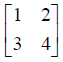NCERT Solutions:NCERT Solutions for Class 12 Maths Determinants Exercise 4.5: Ques No 2.NCERT Solutions: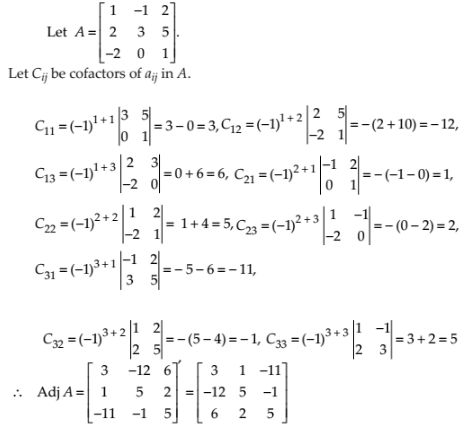Verify A (adj A) = (adj A) A = |A| I in Exercises 3 and 4.

NCERT Solutions for Class 12 Maths Determinants Exercise 4.5: Ques No 3.NCERT Solutions:NCERT Solutions for Class 12 Maths Determinants Exercise 4.5: Ques No 4.NCERT Solutions: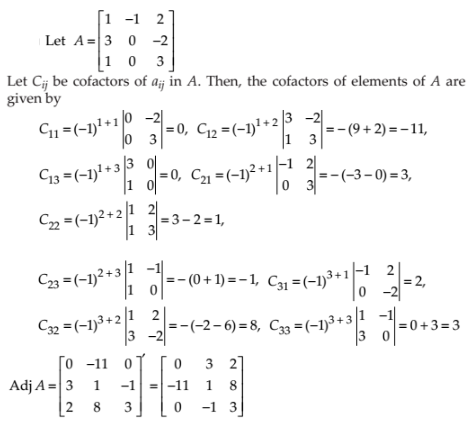Find the inverse of each of the matrices (if it exists) given in Exercises 5 to 11.

NCERT Solutions for Class 12 Maths Determinants Exercise 4.5: Ques No 5.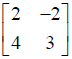NCERT Solutions: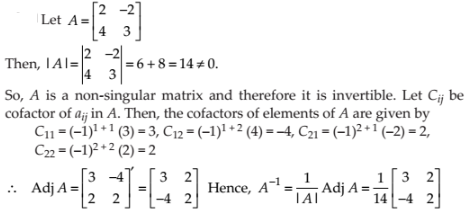NCERT Solutions for Class 12 Maths Determinants Exercise 4.5: Ques No 6.NCERT Solutions: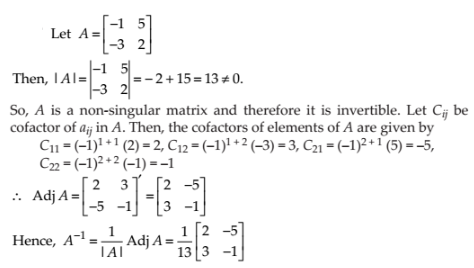NCERT Solutions for Class 12 Maths Determinants Exercise 4.5: Ques No 7.NCERT Solutions:NCERT Solutions for Class 12 Maths Determinants Exercise 4.5: Ques No 8.NCERT Solutions:NCERT Solutions for Class 12 Maths Determinants Exercise 4.5: Ques No 9.NCERT Solutions: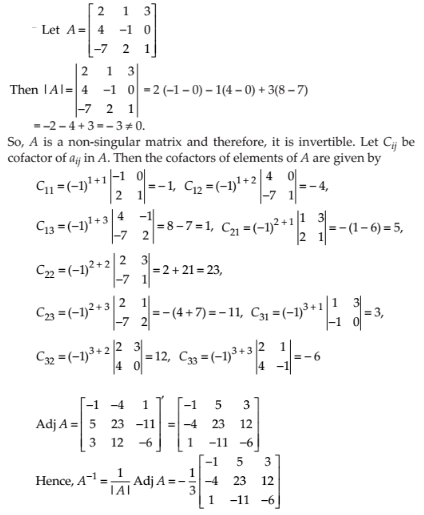NCERT Solutions for Class 12 Maths Determinants Exercise 4.5: Ques No 10.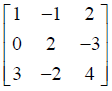NCERT Solutions: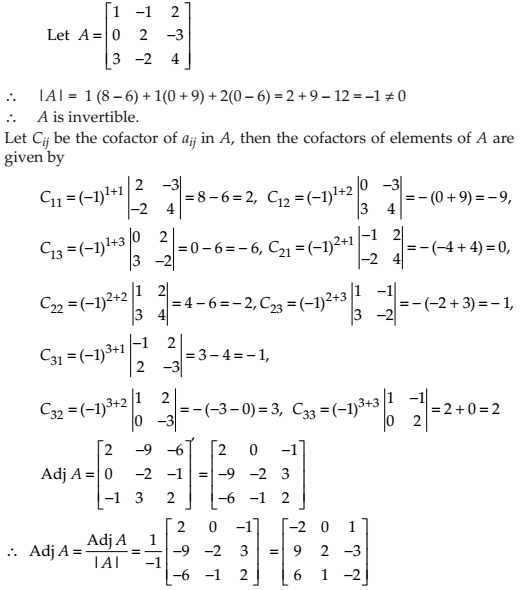NCERT Solutions for Class 12 Maths Determinants Exercise 4.5: Ques No 11.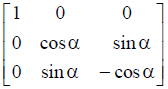NCERT Solutions: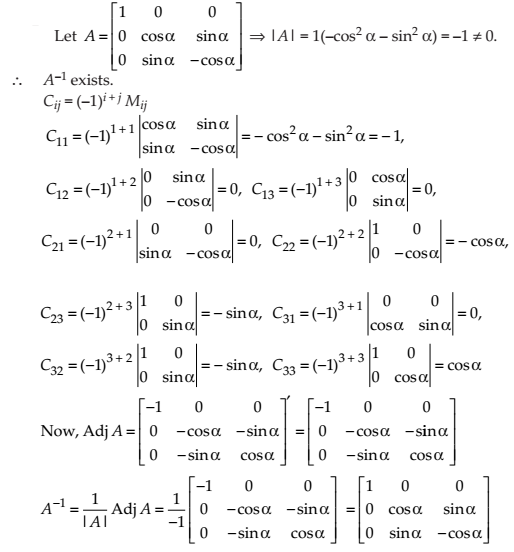NCERT Solutions for Class 12 Maths Determinants Exercise 4.5: Ques No 12.

Let A =and B =. Verify that (AB)–1 = B–1A–1.

NCERT Solutions:NCERT Solutions for Class 12 Maths Determinants Exercise 4.5: Ques No 13.

If A =, Show that A2 – 5A + 7I = O. Hence find A–1.

NCERT Solutions: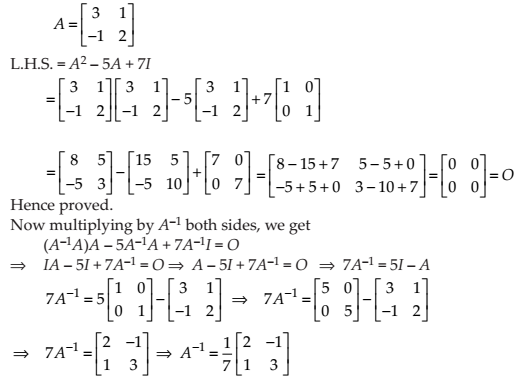NCERT Solutions for Class 12 Maths Determinants Exercise 4.5: Ques No 14.

For the matrix A =, find the numbers a and b such that A2 + aA + bI = O.

NCERT Solutions: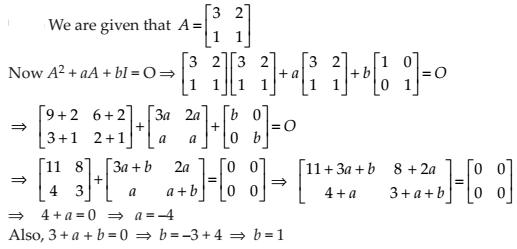NCERT Solutions for Class 12 Maths Determinants Exercise 4.5: Ques No 15.

For the matrix A =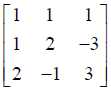, Show that A3– 6A2 + 5A + 11 I = O. Hence, find A–1.

NCERT Solutions: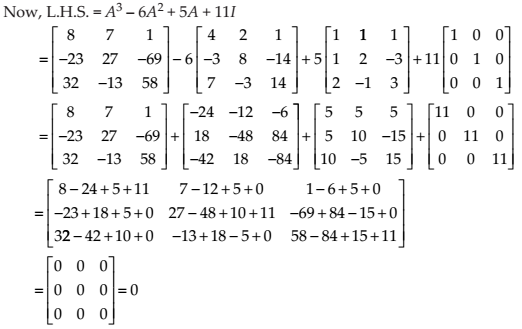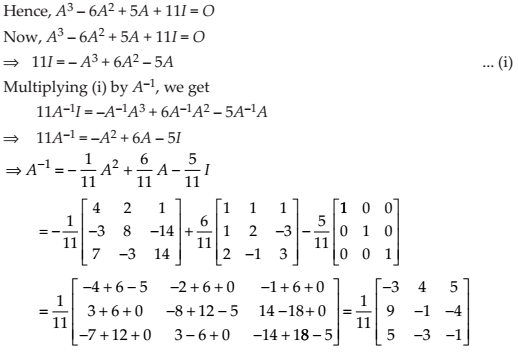NCERT Solutions for Class 12 Maths Determinants Exercise 4.5: Ques No 16.

If A =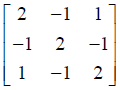, Verify that A3 – 6A2 + 9A – 4I = O and hence find A–1.

NCERT Solutions: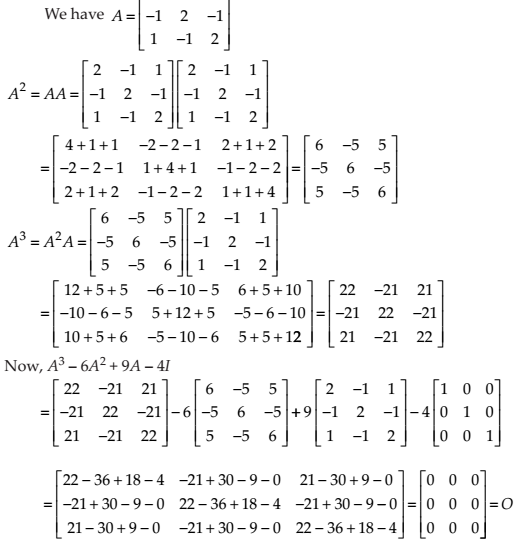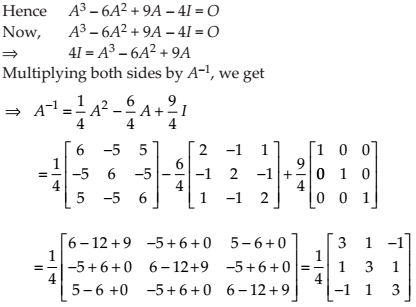NCERT Solutions for Class 12 Maths Determinants Exercise 4.5: Ques No 17.

Let A be a nonsingular square matrix of order 3 × 3. Then |adj A| is equal to

(A) |A|

(B) |A|2

(C) |A|3

(D) 3|A|

NCERT Solutions:

(B) For any n x n matrix A, det(adj A) = |adj A| = |A|n-1. (It holds for singular and non-singular matrices.)

NCERT Solutions for Class 12 Maths Determinants Exercise 4.5: Ques No 18.

If A is an invertible matrix of order 2, then det (A–1) is equal to

(A) det (A)

(B) 1/det (A)

(C) 1

(D) 0

NCERT Solutions:

(B) If A is an invertible matrix of order 2, then AA-1 = I2 = A-1A, where I2 is an identity matrix of order 2. So, det(AA-1) = det(I)

So, detA.detA-1 = 1,

error: Content is protected !!